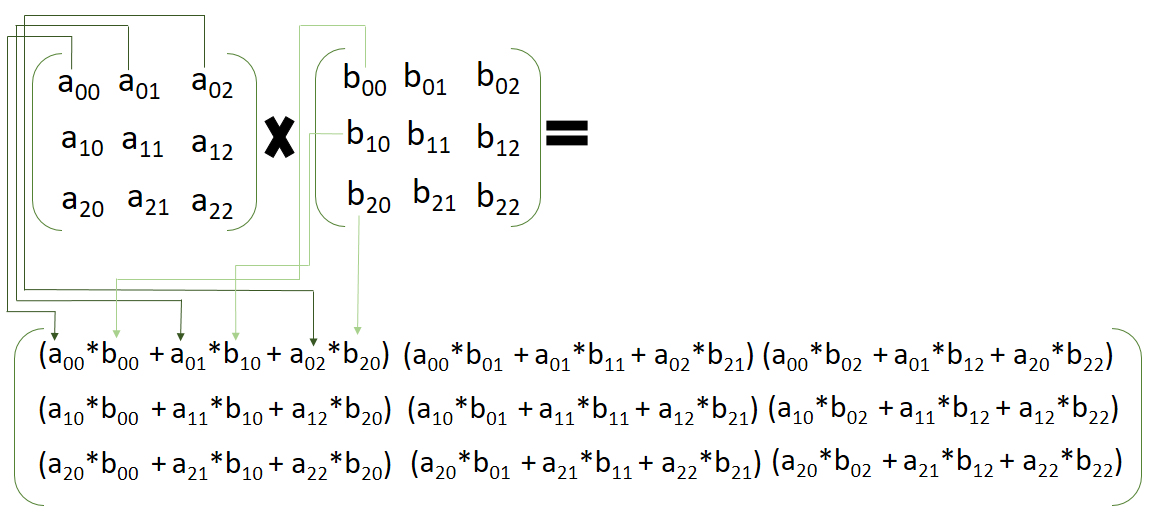# How to Multiply Matrices

• Last Updated : 13 Jan, 2021

The knowledge of matrices is necessary for various branches of mathematics. Matrices are one of the most powerful tools in mathematics. In this article, we discuss the Multiplication of Matrices by matrices. Matrix multiplication is a very simple thing in mathematics. If do multiply with a number then just multiply the number with all the elements present in the matrix. But if we multiply a matrix with another matrix then we must see some rules.

Rules:

We can multiply two matrices if the number of rows in the 1st matrix is the same as the number of columns in the 2nd matrix, otherwise, we cannot apply multiplication between the matrices.

1. Let’s say a matrix of size 2×3 and another matrix is of size 3×2, then we can apply the multiplication between those matrices because the number of columns and rows are the same in both the matrices. And the resultant matrix will be of 2×2
2. Let’s say a matrix of size 3×3 and another matrix is of size 4×2, then we cannot apply the multiplication between those matrices because the number of columns and rows are not the same in both the matrices.

### Multiplying Matrices by Matrices

Let’s say 2 matrices of 3×3 have elements a[i, j] and b[i, j] respectively. The multiplication will be like the below image:There is some rule,

1. Take the first matrix’s 1st row and multiply the values with the second matrix’s 1st column. Don’t multiply the rows with the rows or columns with the columns.
2. In 1st iteration, multiply the row value with the column value and sum those values. Here in this picture, a[0, 0] is multiplying with b[0, 0], then the 2nd value of the 1st column of 1st matrix a[1, 0] is multiplying with 2nd value of the 1st row of the 2nd matrix b[0,1].  Similarly, all the values are multiplying.
3. Then after one iteration all the production values are sum up together and placed as the elements of the resultant matrix as the picture shows.

### Examples

Question 1. LetandFind AxB?

Solution:Question 2. Letand. Find AxB?

Solution:Question 3. Let,and. Find (AB + AC)?

Solution:Now calculate  (AB + AC)Question 4. Let, and, Then find the value of p?

Solution:

First, calculate A2,GivenBy placing the value of A2 in the equation, we getNow, we can write,

8 = 2p and, -8 = -2p

So, p = 4

The value of p is 4.

My Personal Notes arrow_drop_up